Past Issues

× COMMENTARYThe 'Green' Chemistry Challenge
The Green Chemistry Challenge Awards recognize scientific solutions to environmental…
COVER STORYIN THE NEWS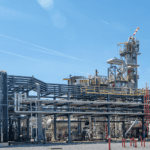Business News: July 2022
Plant Watch Solvay completes PVDF capacity expansion in Changshu June…
NEWSFRONTSTraversing a Data-Powered Supply Chain
Transporting chemical products across global supply chains is complex and…
CHEMENTATORPolymer-coal composite used for building materials
X-Materials (Orlando, Fla.; www.x-materials.com), the advanced materials division of Semplastics…Boosting methane yields in biogas plants
Biogas plants play an important role in defossilization — anaerobic…Recycling Portland cement — emissions-free
The production of clinker used for making cement continues to…'Symbiotic' electrolyzer runs on waste heat
A new steam-fed electrolyzer aims to bridge the gap between…Chemenator Briefs
Cellulosic ethanol Clariant AG (Muttenz, Switzerland; www.clariant.com) has produced the…
TECHNICAL & PRACTICALTOWER DOCTORFEATURE REPORTFACTS AT YOUR FINGERTIPSSOLIDS PROCESSINGConveyor Selection for Bulk Solids Processes
Use this guide to aid the selection of conveying technologies…
EQUIPMENT & SERVICESAPPLIED TECHNOLOGIESOne-stage Filtration for Silicone Production
Presented here is a fast and flexible solution that removes…
FOCUS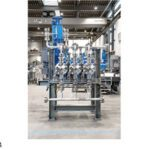Focus on Pumps
Track pump operation with this monitoring system This company has…
SHOW PREVIEWSAchema 2022 Show Preview
Achema (www.achema.de), the global forum for the chemical engineering and…

Processing & Handling

# Fluid Flow

By Department Editor: Kate Torzewski |

## Laminar Pipe Flow

For steady flow in a pipe (whether laminar or turbulent), a momentum balance on the fluid gives the shear stress at any distance from the pipe centerline.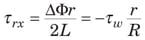In Equation (1), Φ = P + ρ gz. The volumetric flowrate Q can be related to the local shear rate by doing an integration by parts of Equation (2).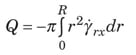Newtonian fluid. For a Newtonian fluid, τ rx = µY rx, which gives the following volumetric flowrate, known as the Hagen-Poiseuille equation.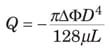It can be written in dimensionless form in Equation (4) with the two terms defined in Equations (5) and (6).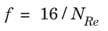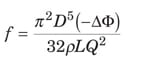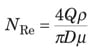Power law. A fluid that follows the power law model obeys the relationship τ rx = – µ(– Y rx) n. This gives the following equation.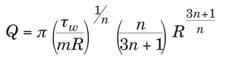Equation (7) can be rearranged into the following dimensionless form.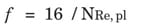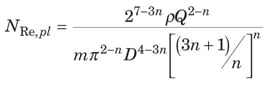Bingham plastic. In this case, there is a solid-like “plug flow” region from the pipe centerline (where τ rx = 0) to the point where – τ rx = τ 0 (that is, at r = r 0 = R x τ 0/ τ w). The result is a flow integral modified from Equation (2). For a Bingham plastic, – τ rx = τ 0 + µ ( Y rx). Using this expression and the modified flow integral, the Buckingham-Reiner Equation (10) is found.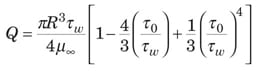The equivalent dimensionless form is given by Equations (11), (12) and (13).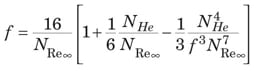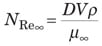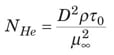## Turbulent Pipe Flow

Since most turbulent flows cannot be analyzed from a purely theoretical perspective, data and generalized dimensionless correlations are used.

Newtonian fluid. The friction factor for a Newtonian fluid in turbulent flow is a function of both N Re and the pipe relative roughness, ε /D, which can be read off the Moody diagram [ 5]. The turbulent part of the Moody diagram (for N Re > 4,000) is accurately represented by the Colebrook equation (14).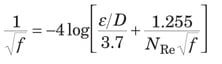When N Re is very large, the friction factor depends only on ε /D. This condition is noted with f T as the “fully turbulent” friction factor in Equation (15).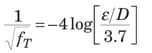The Churchill Equation [ 2] represents the entire Moody diagram, from laminar, through transition flow, to fully turbulent flow. It is presented here as Equations (16), (17), and (18).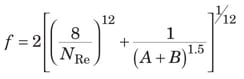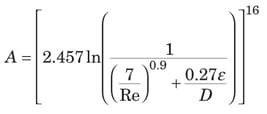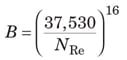Power law. For a power-law fluid, the friction factor depends only upon Equation (9) and the flow index, as represented by Equations (19) – (25) [ 3].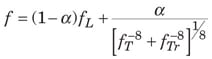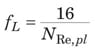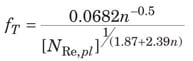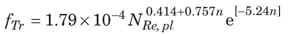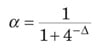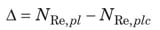The value of N Re where transition from laminar to turbulent flow occurs ( N Re,plc) is given by Equation (25).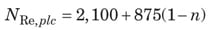Bingham plastic. For the Bingham plastic, f T is solely a function of N Re and N He, as represented by Equations (26) – (29).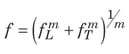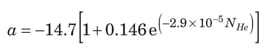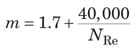## Definitions

Newtonian fluid. A fluid is known to be Newtonian when shear stresses associated with flow are directly proportional to the shear rate of the fluid

Power law fluid. A structural fluid has a structure that forms in the undeformed state, but then breaks down as shear rate increases. Such a fluid exhibits “power law” behavior at intermediate shear rates

Bingham plastic fluid. A plastic is a material that exhibits a yield stress, meaning that it behaves as a solid below the stress level and as a fluid above the stress level

## Nomenclature

a Dimensionless parameter

A Dimensionless parameter

B Dimensionless parameter

D Diameter, m

f Fanning friction factor, dimensionless

f L Laminar friction factor, dimensionless

f T Fully turbulent friction factor, dimensionless

f Tr Transition friction factor, dimensionless

g Gravitational acceleration, m/s 2

L Length of cylinder or pipe, m

m Consistency coefficient, (N)(s)/m 2

n Power law fluid flow index, dimensionless

N He Hedstrom number, dimensionless

N Re Reynolds Number, dimensionless

N Re,pl Power law Reynolds Number, dimensionless

N Re,plc Power law Reynolds Number at transition from laminar to turbulent flow, dimensionless

N Re∞ Bingham-plastic Reynolds Number, dimensionless

P Pressure, Pa

Q Volumetric flowrate, m 3 /s

r Radial position in a pipe or a cylinder, m

R Pipe or cylinder radius, m

V Velocity, m/s

z Vertical elevation above a horizontal reference plane, m

α Dimensionless parameter

Y rx Shear rate in tube flow, s – 1

ε Wall roughness, m

µ Newtonian viscosity, Pa – s

µ Bingham Plastic limiting viscosity, Pa – s

ρ Density, kg/m 3

τ 0 Yield stress, N/m 2

τ rx Stress due to force in x direction acting on r surface, N/m 2

τ w Stress exerted by fluid on tube wall, N/m 2

Φ Flow potential, P + ρ gz, Pa

âˆ†Φ Increase in flow potential, Pa

## References

1. Darby, R., Take the Mystery Out of Non-Newtonian Fluids, Chem. Eng., March 2001, pp. 66 – 73.

2. Churchil, S. W., Friction Factor Equation Spans all Fluid-Flow Regimes, Chem. Eng., November 1997, p. 91.

3. Darby, R., and Chang, H. D., A Generalized Correlation for Friction Loss in Drag-reducing Polymer Solutions, AIChE J., 30, p. 274, 1984.

4. Darby, R., and Chang, H. D., A Friction Factor Equation for Bingham Plastics, Slurries and Suspensions for all Fluid Flow Regimes, Chem. Eng., December 28, 1981, pp. 59 – 61.

5. Darby, R., “Fluid Mechanics for Chemical Engineers,” Vol. 2, Marcel Dekker, New York, N.Y., 2001.

Related Content
Focus: Pipes, Tubes and Fittings
Antimicrobial tubing reduces the risk of bacterial transfer Clearflo Ag-47 antimicrobial tubing (photo) is now available in an additional seven…

Chemical Engineering publishes FREE eletters that bring our original content to our readers in an easily accessible email format about once a week.
Subscribe NowJob Listings